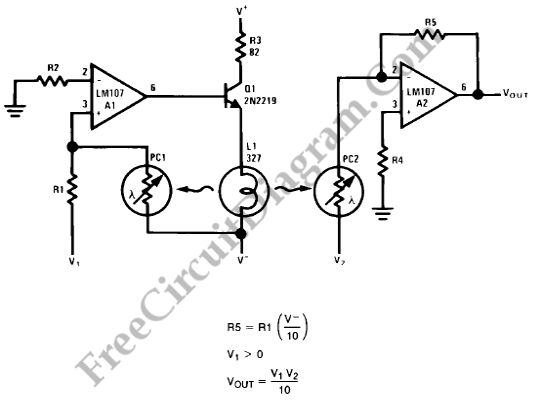# Analog MultiplierThe figure below shows a simple embodiment of the analog multiplier. This circuit provides three quadrant analog multiplication which is relatively temperature insensitive and which is not subject to the bias current errors which is plague most multipliers and circumvents many of the problems associated with the log-antilog circuit.By considering A2 as a controlled gain amplifier, amplifying V2, whose gain is dependent on the ratio of the resistance of PC2 to R5 and by considering A1 as a control amplifier which establishes the resistance of PC2 as function of V1, circuit operation may be used. It is seen that Vout is a function of both V1 and V2 in that way.

Drive for the lamp L1 is provided by the control amplifier(A1). L1 is driven by A1 until the current to the summing junction from the negative supply through PC1 is equal to the current to the summing junction from V1 through R1 when an input voltage (V1) is present. This forces the resistance of PC1 to a value proportional to R1 and the ratio of V1 to V- since the negative supply voltage is fixed. L1 also illuminates PC2 and causes PC2 to have a resistance equal to PC1 if the photoconductors are matched.

The controlled amplifier (A2) behaves as an inverting amplifier whose gain is equal to the ratio of the resistance of PC2 to R5. Vout becomes simply the product of V1 and V2 if R5 is chosen equal to the product of R1 and V-. To provide any required output scale factor, R5 may be scaled in powers of ten.

Since the T.C. of resistance is related to resistance match for cells of the same geometry, PC1 and PC2 should be matched for best tracking over temperature. Varying the value of R5 as a scale factor adjustment is used to compensate small mismatches. The photoconductive cells should receive equal illumination from L1, a convenient method is to mount the lamp midway between them. Controlled spacing and a thermal bridge between the two cells to reduce differences in cell temperature is provided by this mounting method. To the use of FET’s or other devices to meet special resistance or environment requirements, we can extend this technique.

An inverting output whose magnitude is equal to one-tenth the product of the two analog inputs is given on this circuit. Positive value is restricted for input V1 but V2 may assume both positive and negative value. By the lamp time constant, his circuit is restricted to low frequency operation.

To minimize errors due to input offset current as outlined in the section describing the photocell amplifier, R2 and R4 are chosen. To reduce in-rush current when firdt turning on the lamp (L1), R3 is included. [Source: National Semiconductor Application Note]